Python tkinter Canvas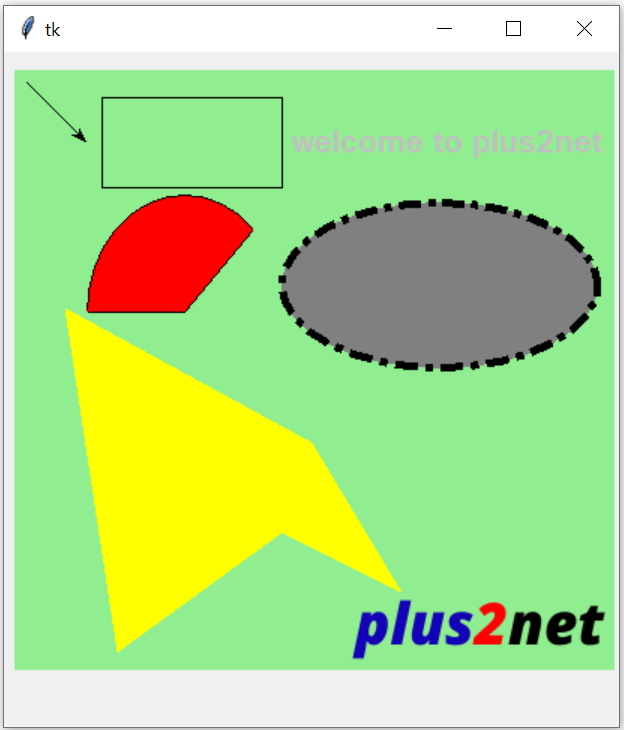Canvas can be used to create graphics by using Lines, rectangles , Arcs etc. It can be used to hold different types of widgets.

X - Horizontal coordinates
Y - Vertical coordinates

Basics of Python Tkinter

Tkinter canvas to add items like line, text , rectangle , oval, polygon, arc and Images with options
import tkinter as tk
my_w = tk.Tk()

my_c = tk.Canvas(my_w,width=200,height=200)
my_c.pack()

my_w.mainloop()
We can create different type of shapes to place over a canvas.

create_text

import tkinter as tk
my_w = tk.Tk()

my_c = tk.Canvas(my_w,width=350,height=150)
my_c.pack()

my_c.create_text(175,40,fill='#c0c0c0',font="Times 22  bold",text="Welcome to plus2net.com")
my_w.mainloop()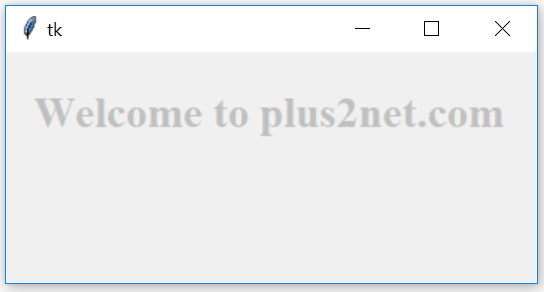create_line

import tkinter as tk
my_w = tk.Tk()

my_c = tk.Canvas(my_w,width=100,height=100)
my_c.pack()

x1=0
y1=50
x2=90
y2=50
my_c.create_line(x1,y1, x2,y2, fill="#ff00ff")

my_w.mainloop()
We can add width to our line
my_c.create_line(x1,y1, x2,y2, fill="#ff00ff",width=5)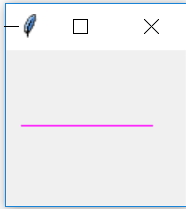create_rectangle

import tkinter as tk
my_w = tk.Tk()

my_c = tk.Canvas(my_w,width=200,height=200)
my_c.pack()
my_c.create_rectangle(80,80,110,110,fill='#c0c0c0')

my_w.mainloop()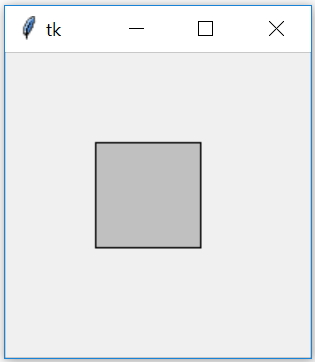create_oval

We will crate one oval using create_oval
import tkinter as tk
my_w = tk.Tk()

my_c = tk.Canvas(my_w,width=150,height=150)
my_c.pack()
my_c.create_oval(25,25,125,125,fill='#c0c0c0')

my_w.mainloop()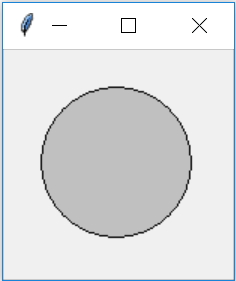Create one Circle by using create_oval
import tkinter as tk
my_w = tk.Tk()

my_c = tk.Canvas(my_w,width=200,height=200)
my_c.pack()

def my_circle(my_canvas,x,y,r):
my_id=my_canvas.create_oval(x-r,y-r,x+r,y+r,fill='#c0c0c0')
return my_id

my_circle(my_c,60,60,15)
#my_c.create_oval(60,60,130,130,fill='#c0c0c0')
my_w.mainloop()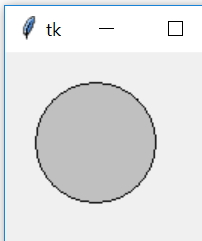create_image

import tkinter as tk
my_w = tk.Toplevel()
from PIL import Image, ImageTk

my_c = tk.Canvas(my_w,width=200,height=200)
my_c.pack()
#image = Image.open("icon-dwn.png")
f_name = tk.PhotoImage(file='icon-dwn.png')
my_img = my_c.create_image(50, 50,  image=f_name)

my_w.mainloop()

create_arc

import tkinter as tk
my_w = tk.Tk()

my_c = tk.Canvas(my_w,width=150,height=150)
my_c.pack()

my_c.create_arc(10,10,130,130,start=15,extent=160,fill='#c0c0c0')
my_w.mainloop()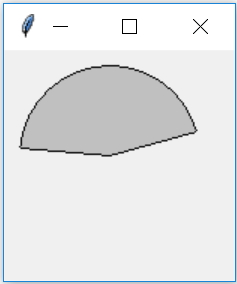create_polygon

import tkinter as tk
my_w = tk.Tk()

my_c = tk.Canvas(my_w,width=150,height=150)
my_c.pack()

my_c.create_polygon(5,40,15,120,130,70,35,5,fill='#c0c0c0')
my_w.mainloop()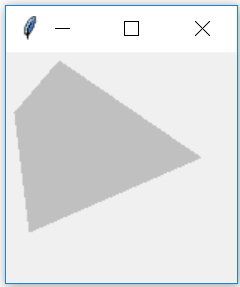Options

 fill Colour used to fill the shape width Line width of the item ( or its outline) outline Colour of the outline ( rectangle , Oval etc. ) dash Draw dashed line instead of solid Line width of the item ( or its outline). alternates short and long pixels. ( 2,5,210) outline Colour of the outline ( rectangle , Oval etc. ) stipple pattern fill instead of solid fill colour. gray75,gray50 etc.. state normal, disabled or hidden . Default value is normal activefill Colour when active ( mouse pointer is over the item ) activeoutline Colour of the outline when active ( Mouse is over the item ) activedash Same as dash ( above ) when active ( Mouse is over it ) activewidth Same as width ( above ) when active ( Mouse is over it ) disableddash When the item is disabled ( state=disabled) disabledfill disabledoutline disabledoutlinestipple disabledstipple disabledwidthimport tkinter as tk
my_w = tk.Tk()
width,height=410,410 # set the variables
d=str(width)+"x"+str(height+40)
my_w.geometry(d)
c1 = tk.Canvas(my_w, width=width-10, height=height-10,bg='lightgreen')
c1.create_text(290,50,fill='#c0c0c0',font='Time 16 bold',text='welcome to plus2net')
my_rect=c1.create_rectangle(60, 20, 180, 80)
c1.create_oval(180, 90, 390, 200,fill='gray',dash=(25,5,1,10),activedash=(50,10),stipple='gray75',width=5,activestipple='gray25')
c1.create_line(10, 10, 50, 50,width=1,arrow='last')
c1.create_arc(50, 85, 180, 240, start=45, extent=135, fill="red")
c1.create_polygon(35,160, 70, 390, 180, 310,260, 350, 200, 250, fill='yellow')
f_name=tk.PhotoImage(file='D:\\top2.png')
my_img=c1.create_image(315,375,image=f_name)
#c1.delete(my_rect)
my_w.mainloop()

Subscribe

* indicates required
Subscribe to plus2netplus2net.com